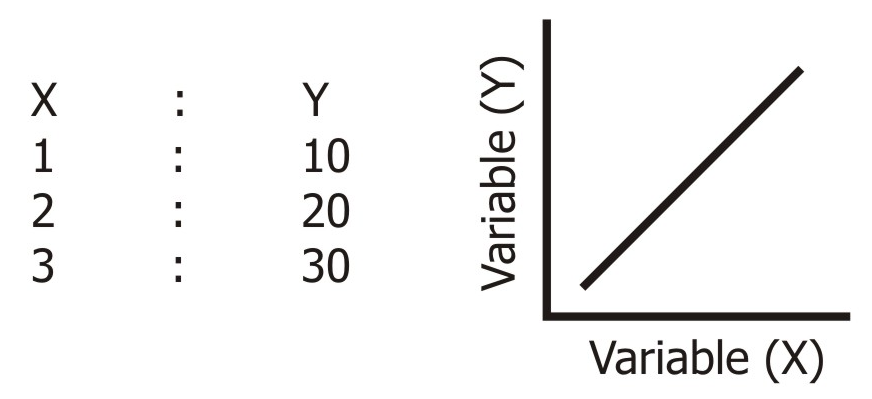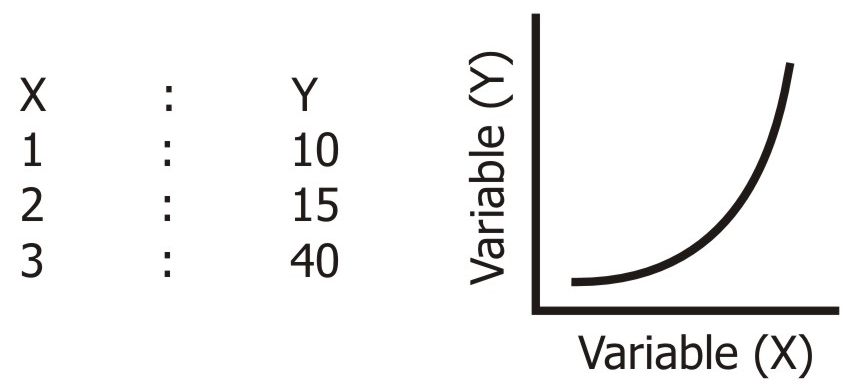# Difference between Linear and Curvilinear Correlation

## Meaning of Linear Correlation

Linear correlation is referred to as the measure of relationship between two random variables with values ranging from -1 and 1. It is proportional to covariance and can be interpreted in the same way as covariance.

Linear correlation is also said to be based on a straight-line relationship between two random variables.

## Meaning of Curvilinear Correlation

Non-linear or curvilinear correlation is said to occur when the ratio of change between two variables is not constant. It can happen that as the value of one variable increases, the value of another variable also increases. This will happen till a certain point, after which the increase in value of one variable will result in the decrease in value of the other variable.

The graphical representation of a curvilinear correlation is like an inverted U.

## Linear and Curvilinear Correlation

 Positive Correlation Negative Correlation Two variables are said to have a positive correlation when they move in the same direction i.e. change occurs in them in the same direction. In positive correlation, the increase in value of one variable results in an increase in the value of another variable. Example, The area under cultivation and agricultural production The use of manure and the increase in output The expenditure on advertisement and the increase in sales Two variables are said to have a negative correlation when they move in the opposite direction i.e. change occurs in them in the opposite direction. In negative correlation, the increase in value of one variable results in decrease in the value of another variable. Example, The price of onions and the  demand of onions The production of vegetables and the prices of vegetables The time spent on video games and the marks in exams

 Linear Correlation Curvilinear Correlation There exists a linear correlation if the ratio of change in the two variables is constant. ●     If we plot these coordinates on a graph, we will get a straight line.There exists a curvilinear correlation if the change in the variables is not constant. ●     If we plot these coordinates on a graph, we will get a curve.Simple, Partial, and Multiple Correlations Simple correlation: When we consider only two variables and check the correlation between them, it is termed as simple correlation. For example, the radius and circumference of a circle. Multiple correlation: When we consider three or more variables for correlation, it is termed as multiple correlation. For example, the price of cola drink, temperature, income, and the demand for cola. Partial correlation: When one or more variables are kept constant and the relationship is studied between the others, it is termed as partial correlation. For example, if we keep the price of cola constant and check the correlation between temperature and the demand for cola, it is a partial correlation.

This concept was about the difference between linear and curvilinear correlations for the Class 11 commerce students. Stay tuned for questions papers, sample papers, syllabus, and relevant notifications on our website.

Also check:

#### 1 Comment

1. Shreyansh sheth

I have understood the difference, thanks